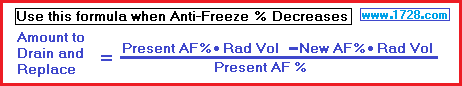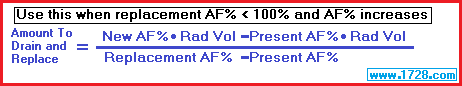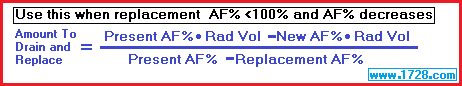Scroll down for instructions.

This calculator was written to solve the typical "radiator problem" encountered in just about any algebra class or textbook.
The most common calculation (as in Problem 1) involves determining how much liquid to drain (and replace with 100% antifreeze) to achieve a higher antifreeze percentage in the radiator.

 Problem 1 A 28 liter car radiator has a 50% antifreeze solution. To prepare for winter driving this must be increased from 50% to 80% antifreeze. How much liquid should be drained and replaced with 100% antifreeze in order to have an 80% mixture? To solve this problem you will need this formula:(In all these formulas, use percentages divided by 100. So 50% would be calculated as .50) Amount to Drain and Replace = [(.80 * 28) -(.50 * 28)] ÷ (1 -.5) Amount to Drain and Replace = (22.4 - 14) ÷ .5 Amount to Drain and Replace = 8.4 ÷ .5 Amount to Drain and Replace = 16.8 Using the Calculator: If "Replacement = 100%" hasn't already been selected, click that button Then enter: 28 for radiator volume 50 for present antifreeze percentage 80 for new antifreeze percentage Click "CALCULATE" and your answer should be 16.8

 Problem 2 A 24 liter car radiator has a 75% antifreeze solution. To prepare for summer driving, the solution must be reduced to 50%. How much of the solution needs to be drained and replaced with water to achieve this 50% mixture? When, the anti-freeze percentage decreases, a different formula is required.Amount to Drain and Replace = [(.75 * 24) -(.50 * 24)] ÷ .75 Amount to Drain and Replace = (18 -12) ÷ .75 Amount to Drain and Replace = 6 ÷ .75 Amount to Drain and Replace = 8 Using the Calculator: If "Replacement = 100%" hasn't already been selected, click that button Then enter: 24 for radiator volume 75 for present antifreeze percentage 50 for new antifreeze percentage Click "CALCULATE" and your answer should be 8

Problem 3
A 16 liter car radiator contains a 50% antifreeze mixture which needs to be increased to 75% antifreeze for winter driving.
How much liquid should be drained and replaced with 90% antifreeze in order to have a 75% mixture?

To solve this problem you will need this formula:Amount to Drain and Replace = [(.75 * 16) -(.50 * 16)] ÷ (.90 -.50)

Amount to Drain and Replace = (12 -8) ÷ (.4)

Amount to Drain and Replace = 4 ÷ .4

Amount to Drain and Replace = 10

Using the Calculator:
Click the "Replacement < 100%" button

Then enter:
50 for present antifreeze percentage
75 for new antifreeze percentage
90 for replacement antifreeze percentage

Problem 4
A 20 quart car radiator contains an 80% antifreeze mixture which needs to be decreased to a 50% antifreeze mixture for summer driving.
How much liquid should be drained and replaced with 10% antifreeze in order to have a 50% mixture?

To solve this problem you will need this formula:Amount to Drain and Replace = [(.80 * 20) -(.50 * 20)] ÷ (.80 -.10)

Amount to Drain and Replace = (16 -10) ÷ .7

Amount to Drain and Replace = 6 ÷ .7

Amount to Drain and Replace = 8.5714

Using the Calculator:
Click the "Replacement < 100%" button

Then enter: